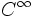# Difference between revisions of "Differential manifold"

Jump to: navigation, search

## Definition

### Definition in terms of atlases

A differential manifold or smooth manifold is the following data:

• A topological space$M$
• An atlas of coordinate charts on$M$ to$\R^n$ (in other words an open cover of$M$ with homeomorphisms from each member of the open cover to open sets in$\R^n$

satisfying the compatibility condition: the transition function between any two coordinate charts of the atlas is a diffeomomorphism of open subsets of$\R^n$.

By diffeomorphism, we here mean a$C^{\infty}$ map with a$C^{\infty}$ inverse.

upto the following equivalence:

Two atlases of coordinate charts on a topological space define the same differential manifold structure if given any coordinate chart in one and any coordinate chart in the other, the transition function between them is a diffeomorphism.

### Definition in terms of sheaves

A differential manifold or smooth manifold is the following data:

• A topological space$M$
• A subsheaf of the sheaf of continuous functions from$M$ to$\R$, which plays the role of the sheaf of differentiable functions

Fill this in later